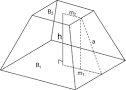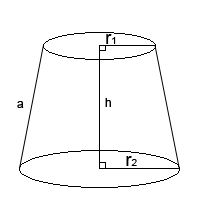## How to Calculate and Solve for the Height and Volume of a Square Pyramid Frustum | Nickzom CalculatorThe image above is a square pyramid frustum.

To compute the volume of a square pyramid frustum, three essential parameters are needed and this parameters are base side length (a), top side length (b) and height (h).

The formula for calculating the volume of a square pyramid frustum:

V = h(a² + ab + b²)3

Where;
V = Volume of a square pyramid frustum
a = Base side length of the square pyramid frustum
b = Top side length of the square pyramid frustum
h = Height of the square pyramid frustum

Let’s solve an example
Find the volume of a square pyramid frustum when the base side length is 10 cm with a top side length of 12 cm and a height of 20 cm.

This implies that;
a = Base side length of the square pyramid frustum = 10 cm
b = Top side length of the square pyramid frustum = 12 cm
h = Height of the square pyramid frustum = 20 cm

V = h(a² + ab + b²)3
V = 20(10² + (10 x 12) + 12²)3
V = 20(100 + (120) + 144)3
V = 20(364)3
V = 72803
V = 2426.6

Therefore, the volume of the square pyramid frustum is 2426.6 cm3.

Calculating the Height of a Square Pyramid Frustum when the Volume, Top Side Length and Base Side Length of the Square Pyramid Frustum is Given.

h = 3V / (a2 + ab + b2)

Where;
h = Height of the square pyramid frustum
V = Volume of the square pyramid frustum
a = Base side length of the square pyramid frustum
b = Top side length of the square pyramid frustum

Let’s solve an example;
Find the height of a square pyramid frustum when the volume is 32 cm3, base side length is 14 cm and top side length is 18 cm.

This implies that;
V = Volume of the square pyramid frustum = 32 cm3
a = Base side length of the square pyramid frustum = 14 cm
b = Top side length of the square pyramid frustum = 18 cm

h = 3V / (a2 + ab + b2)
h = 32 x 3 / (142 + (14 x 18) + 182)
h = 96 / (196 + 252 + 324)
h = 96 / 772
h = 0.124

Therefore, the height of the square pyramid frustum is 0.124 cm.

## How to Calculate and Solve for the Total Surface Area of a Conical Frustum | The Calculator EncyclopediaThe image above is a conical frustum.

To compute the total surface area of a conical frustum requires three essential parameters which are the radius of the lower base (R), radius of the upper base (r) and the height (h).

The formula for calculating the total surface area of a conical frustum;

A = π[R² + r² + (R + r)√((R – r)² + h²)]

Where;
A = Total surface area of the conical frustum
R = Radius of the lower base
r = Radius of the upper base
h = Height of the conical frustum

Let’s solve an example;
Find the total surface area of the conical frustum when the radius of the upper base is 11 cm, radius of the lower base is 17 cm and the height is 30 cm.

This implies that;
r = Radius of the upper base = 11 cm
R = Radius of the lower base = 17 cm
h = Height of the conical frustum = 30 cm

A = π[R² + r² + (R + r)√((R – r)² + h²)]
A = π[17² + 11² + (17 + 11)√((17 – 11)² + 30²)]
A = π[289 + 121 + (28)√((6)² + 900)]
A = π[289 + 121 + (28)√(36+ 900)]
A = π[289 + 121 + (28)√(936)]
A = π[289 + 121 + (28)(30.59)]
A = π[289 + 121 + 856.63]
A = π[1266.63]
A = 3979.25

Therefore, the total surface area of the conical frustum is 3979.25 cm².